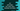# C# program to find the days, hours, minutes, and seconds between two dates## C# program to print the Days, hours, minutes, seconds between two dates:

In this post, we will learn how to print the days, hours, minutes, and seconds between two DateTime objects in C#. DateTime structure is used to store date-time in C#. We can create any date-time object with predefined date and time or we can create one object with the current date and time.

Finding out the difference between two DateTime objects is easy. We can find the difference and it returns one TimeSpan object. TimeSpan object provides different properties those can be used to get the days, hours, minutes and seconds value for that time difference. It makes the process easier and we don’t have to re-calculate anything.

### How to create a DateTime object with different date-time:

We have different ways to create a DateTime object. In this example, we will use the Parse method. For example, the below code snippet creates one DateTime object with the provided info:

``DateTime.Parse("01/22/2021 10:10:10 PM");``

It takes the date-time string and creates the DateTime object. Our program will create two DateTime objects with two different date-time strings.

## C# program to find the date difference:

This program will first create two DateTime objects. It will then find the difference between these objects and print the difference in days, hours, minutes, and seconds.

To find the difference between two DateTime objects, we can either use Subtract method or - . Both gives the same result. We can save the difference in a TimeSpan object.

TimeSpan object provides different properties like:

• Days: It gives the days value in the object.
• Hours: It gives the hours value in the object.
• Minutes: It gives the minutes value in the object.
• Seconds: It gives the seconds value in the object.
• Milliseconds: It gives the milliseconds value in the object.

For this example, I will not use Milliseconds. Using these properties, we can destruct the time.

Below is the complete example:

``````using System;

public class Program
{
public static void Main()
{
DateTime firstDate = DateTime.Parse("01/22/2021 10:10:10 PM");
DateTime secondDate = DateTime.Parse("01/25/2021 12:30:30 AM");

TimeSpan dateDifference = secondDate - firstDate;

Console.WriteLine("Difference : "+dateDifference.Days+" days,"+dateDifference.Hours+" hours, "+dateDifference.Minutes+" minutes and "+dateDifference.Seconds+" seconds");
}
}``````

If you run this program, it will print the below output:

``Difference :2 days,2 hours, 20 minutes and 20 seconds``

Here,

• firstDate is holding the first date-time values and secondDate is holding the second date-time values.
• We are finding the difference by using -, which is a TimeSpan object.
• The last line is only printing the properties of the TimeSpan object dateDifference.

Note that, we can also use Subtract to find the difference between two DateTime objects as like below:

``TimeSpan dateDifference = secondDate.Subtract(firstDate);``

It will give similar output.